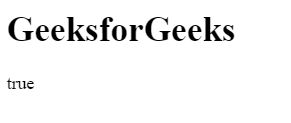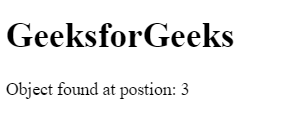# How to check if an array includes an object in JavaScript?

• Last Updated : 20 Jul, 2021

There are various methods to check an array includes an object or not.
Using includes() Method: If array contains an object/element can be determined by using includes() method. This method returns true if the array contains the object/element else return false.
Syntax:

`array.includes( element/object, startingPosition )`

Example:

## html

 ` ` `<``html``> ` ` `  `<``head``> ` `    ``<``title``> ` `        ``JavaScript includes() Method ` `    `` ` ` ` ` `  `<``body``> ` ` `  `    ``<``h1``>GeeksforGeeks ` `     `  `    ``<``p` `id``=``"geeks"``> ` ` `  `     `  `    ``` `    ``<``script``> ` `        ``var obj = {"geeks1":10, "geeks2":12} ` `        ``var gfg = ["geeks1", "geeks2", obj]; ` `         `  `        ``/* Use JavaScript includes() method */ ` `        ``var num = gfg.includes(obj, 0); ` `         `  `        ``document.getElementById("geeks").innerHTML ` `                    ``= num; ` `    `` ` ` ` ` `  `         `

Output:Using some() Method: The some() method uses the function for its evaluation and it executes the function once for each element present in the array. If it finds the object/element in the array then it returns true and stop the execution for remaining elements otherwise return false.
Syntax:

`array.some( function(currValue, arrIndex, arrObj), this )`

Example:

## html

 ` ` `<``html``> ` ` `  `<``head``> ` `    ``<``title``> ` `        ``JavaScript includes() Method ` `    `` ` ` ` ` `  `<``body``> ` ` `  `    ``<``h1``>GeeksforGeeks ` `     `  `    ``` `    ``<``script``> ` `     `  `        ``/* Declare an array */ ` `        ``var arr = ["geeks1", "geeks2", "geeks3", ` `                    ``{1:"geeks4", 2:"geeks5"}]; ` `                     `  `        ``var boolVar = arr.some(  ` `            ``value => { return typeof value == "object" } ); ` `             `  `        ``document.write(boolVar); ` `    `` ` ` ` ` `  `                     `

Output:Using filter() Method: The filter() method creates the array of all those elements/objects that passes the checking condition.
Syntax:

`array.filter( function(currValue, arrIndex, arrObj), this )`

Example:

## html

 ` ` `<``html``> ` ` `  `<``head``> ` `    ``<``title``> ` `        ``JavaScript includes() Method ` `    `` ` ` ` ` `  `<``body``> ` ` `  `    ``<``h1``>GeeksforGeeks ` `     `  `    ``<``p` `id``=``"geeks"``> ` ` `  `     `  `    ``` `    ``<``script``> ` `        ``var obj = {"geeks1":10, "geeks2":12} ` `        ``var arr = ["geeks1", "geeks2", "geeks3", obj]; ` `         `  `        ``if(arr.filter(value=> value==obj).length > 0) ` `            ``document.write("true"); ` `        ``else ` `            ``document.write("false"); ` `    `` ` ` ` ` `  `                     `

Output:Using findIndex() Method: The findIndex() method returns the position of searched object/element if it present in the array and stop the execution for rest elements. If the element/object not found then return -1.
Syntax:

`array.findIndex( function(currValue, arrIndex, arrObj), this )`

Example:

## html

 ` ` `<``html``> ` ` `  `<``head``> ` `    ``<``title``> ` `        ``JavaScript includes() Method ` `    `` ` ` ` ` `  `<``body``> ` ` `  `    ``<``h1``>GeeksforGeeks ` `     `  `    ``` `    ``<``script``> ` `     `  `        ``/* Declare an array */ ` `        ``var arr = ["geeks1", "geeks2", "geeks3", ` `                ``{"geeks1":10, "geeks2":12}]; ` `                 `  `        ``var num = arr.findIndex(  ` `            ``value => { return typeof value == "object" } ); ` `             `  `        ``document.write("Object found at position: " + num); ` `    `` ` ` ` ` `  `                     `

Output:JavaScript is best known for web page development but it is also used in a variety of non-browser environments. You can learn JavaScript from the ground up by following this JavaScript Tutorial and JavaScript Examples.

My Personal Notes arrow_drop_up
Recommended Articles
Page :